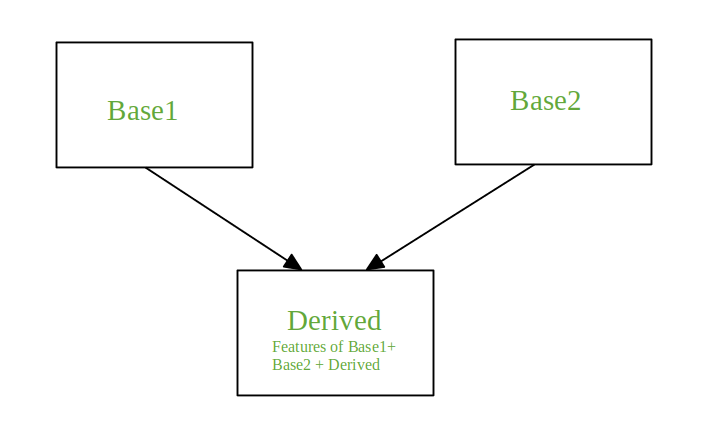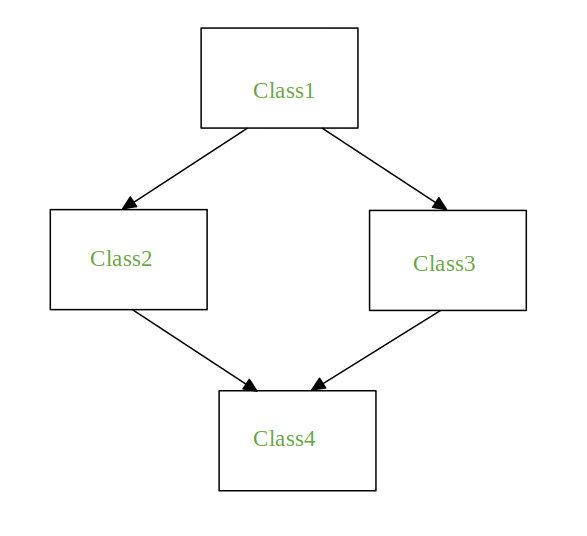# Multiple inheritance in Python

Inheritance is the mechanism to achieve the re-usability of code as one class(child class) can derive the properties of another class(parent class). It also provides transitivity ie. if class C inherits from P then all the sub-classes of C would also inherit from P.

Multiple Inheritance
When a class is derived from more than one base class it is called multiple Inheritance. The derived class inherits all the features of the base case.```Syntax:

Class Base1:
Body of the class

Class Base2:
Body of the class

Class Derived(Base1, Base2):
Body of the class
```

In the coming section we will see the problem faced during multiple inheritance and how to tackle it with the help of examples.

The Diamond ProblemIt refers to an ambiguity that arises when two classes Class2 and Class3 inherit from a superclass Class1 and class Class4 inherits from both Class2 and Class3. If there is a method “m” which is an overridden method in one of Class2 and Class3 or both then the ambiguity arises which of the method “m” Class4 should inherit.

When method is overridden in both classes

 `# Python Program to depict multiple inheritance ` `# when method is overridden in both classes ` ` `  `class` `Class1: ` `    ``def` `m(``self``): ` `        ``print``(``"In Class1"``)  ` `       `  `class` `Class2(Class1): ` `    ``def` `m(``self``): ` `        ``print``(``"In Class2"``) ` ` `  `class` `Class3(Class1): ` `    ``def` `m(``self``): ` `        ``print``(``"In Class3"``)   ` `        `  `class` `Class4(Class2, Class3): ` `    ``pass`    `     `  `obj ``=` `Class4() ` `obj.m() `

Output:

```In Class2
```

Note: When you call `obj.m()` (m on the instance of Class4) the output is In Class2. If Class4 is declared as Class4(Class3, Class2) then the output of obj.m() will be In Class3.

When method is overridden in one of the classes

 `# Python Program to depict multiple inheritance ` `# when method is overridden in one of the classes ` ` `  `class` `Class1: ` `    ``def` `m(``self``): ` `        ``print``(``"In Class1"``)  ` `       `  `class` `Class2(Class1): ` `    ``pass` ` `  `class` `Class3(Class1): ` `    ``def` `m(``self``): ` `        ``print``(``"In Class3"``)     ` `      `  `class` `Class4(Class2, Class3): ` `    ``pass`        ` `  `obj ``=` `Class4() ` `obj.m() `

Output:

```In Class3
```

When every class defines the same method

 `# Python Program to depict multiple inheritance ` `# when every class defines the same method ` ` `  `class` `Class1: ` `    ``def` `m(``self``): ` `        ``print``(``"In Class1"``)  ` `       `  `class` `Class2(Class1): ` `    ``def` `m(``self``): ` `        ``print``(``"In Class2"``) ` ` `  `class` `Class3(Class1): ` `    ``def` `m(``self``): ` `         ``print``(``"In Class3"``)      ` `     `  `class` `Class4(Class2, Class3): ` `    ``def` `m(``self``): ` `        ``print``(``"In Class4"``)    ` ` `  `obj ``=` `Class4() ` `obj.m() ` ` `  `Class2.m(obj) ` `Class3.m(obj) ` `Class1.m(obj) `

Output:

```In Class4
In Class2
In Class3
In Class1
```

The output of the method `obj.m()` in the above code is In Class4. The method “m” of Class4 is executed. To execute the method “m” of the other classes it can be done using the class names.

Now, to call the method m for Class1, Class2, Class3 directly from the method “m” of the Class4 see the below example

 `# Python Program to depict multiple inheritance  ` `# when we try to call the method m for Class1,  ` `# Class2, Class3 from the method m of Class4  ` `  `  `class` `Class1: ` `    ``def` `m(``self``): ` `        ``print``(``"In Class1"``)   ` `      `  `class` `Class2(Class1): ` `    ``def` `m(``self``): ` `        ``print``(``"In Class2"``) ` ` `  `class` `Class3(Class1): ` `    ``def` `m(``self``): ` `        ``print``(``"In Class3"``)      ` `     `  `class` `Class4(Class2, Class3): ` `    ``def` `m(``self``): ` `        ``print``(``"In Class4"``)    ` `        ``Class2.m(``self``) ` `        ``Class3.m(``self``) ` `        ``Class1.m(``self``) ` ` `  `obj ``=` `Class4() ` `obj.m() `

Output:

```In Class4
In Class2
In Class3
In Class1
```

To call “m” of Class1 from both “m” of Class2 and “m” of Class3 instead of Class4 is shown below:

 `# Python Program to depict multiple inheritance ` `# when we try to call m of Class1 from both m of ` `# Class2 and m of Class3 ` ` `  `class` `Class1: ` `    ``def` `m(``self``): ` `        ``print``(``"In Class1"``)    ` `     `  `class` `Class2(Class1): ` `    ``def` `m(``self``): ` `        ``print``(``"In Class2"``) ` `        ``Class1.m(``self``) ` ` `  `class` `Class3(Class1): ` `    ``def` `m(``self``): ` `        ``print``(``"In Class3"``) ` `        ``Class1.m(``self``)    ` `      `  `class` `Class4(Class2, Class3): ` `    ``def` `m(``self``): ` `        ``print``(``"In Class4"``)    ` `        ``Class2.m(``self``) ` `        ``Class3.m(``self``) ` `      `  `obj ``=` `Class4() ` `obj.m() `

Output:

```In Class4
In Class2
In Class1
In Class3
In Class1
```

The output of the above code has one problem associated with it, the method m of Class1 is called twice. Python provides a solution to the above problem with the help of the `super()` function. Let’s see how it works.

The super Function

 `# Python program to demonstrate ` `# super() ` ` `  `class` `Class1: ` `    ``def` `m(``self``): ` `        ``print``(``"In Class1"``) ` ` `  `class` `Class2(Class1): ` `    ``def` `m(``self``): ` `        ``print``(``"In Class2"``) ` `        ``super``().m() ` ` `  `class` `Class3(Class1): ` `    ``def` `m(``self``): ` `        ``print``(``"In Class3"``) ` `        ``super``().m() ` ` `  `class` `Class4(Class2, Class3): ` `    ``def` `m(``self``): ` `        ``print``(``"In Class4"``)    ` `        ``super``().m() ` `      `  `obj ``=` `Class4() ` `obj.m() `

Output:

```In Class4
In Class2
In Class3
In Class1
```

`Super()` is generally used with the `__init__` function when the instances are initialized. The super function comes to a conclusion, on which method to call with the help of the method resolution order (MRO).

#### Method resolution order

In Python, every class whether built-in or user-defined is derived from the object class and all the objects are instances of the class object. Hence, object class is the base class for all the other classes.

In the case of multiple inheritance a given attribute is first searched in the current class if it’s not found then it’s searched in the parent classes. The parent classes are searched in a depth-first, left-right fashion and each class is searched once.

If we see the above example then the order of search for the attributes will be Derived, Base1, Base2, object. The order that is followed is known as a linearization of the class Derived and this order is found out using a set of rules called Method Resolution Order (MRO).

To view the MRO of a class:

• Use the mro() method, it returns a list
Eg. Class4.mro()
• Use the _mro_ attribute, it returns a tuple
Eg. Class4.__mro__

Example:

 `# Python program to demonstrate ` `# super() ` ` `  `class` `Class1: ` `    ``def` `m(``self``): ` `        ``print``(``"In Class1"``) ` ` `  `class` `Class2(Class1): ` `    ``def` `m(``self``): ` `        ``print``(``"In Class2"``) ` `        ``super``().m() ` ` `  `class` `Class3(Class1): ` `    ``def` `m(``self``): ` `        ``print``(``"In Class3"``) ` `        ``super``().m() ` ` `  `class` `Class4(Class2, Class3): ` `    ``def` `m(``self``): ` `        ``print``(``"In Class4"``)    ` `        ``super``().m() ` `      `  `Class4.mro() `

Output:

[<class ‘__main__.Class4’>, <class ‘__main__.Class2’>, <class ‘__main__.Class3’>, <class ‘__main__.Class1’>, <class ‘object’>]
(<class ‘__main__.Class4’>, <class ‘__main__.Class2’>, <class ‘__main__.Class3’>, <class ‘__main__.Class1’>, <class ‘object’>)

My Personal Notes arrow_drop_upCheck out this Author's contributed articles.

If you like GeeksforGeeks and would like to contribute, you can also write an article using contribute.geeksforgeeks.org or mail your article to contribute@geeksforgeeks.org. See your article appearing on the GeeksforGeeks main page and help other Geeks.

Please Improve this article if you find anything incorrect by clicking on the "Improve Article" button below.

Article Tags :

3

Please write to us at contribute@geeksforgeeks.org to report any issue with the above content.